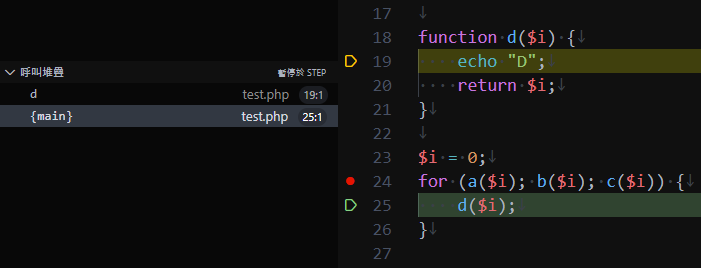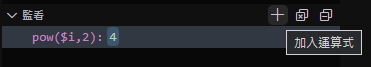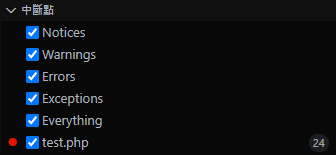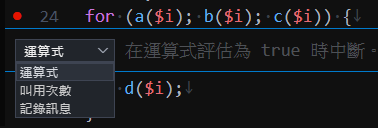DAY 19
0
Software Development

【PHP Telegram Bot】Day19 - 基礎(8)：迴圈、Xdebug

迴圈

while

\$i = 1;
while (2 ** \$i < 1000) {
echo 2 ** \$i, "\n";
\$i++;
}

do while

\$i = 1;
do {
echo 2 ** \$i, "\n";
\$i++;
} while (2 ** \$i < 1000);

for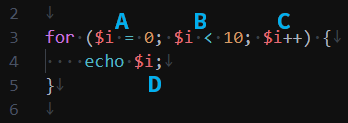1. A：把 0 存進變數 \$i
2. B：邏輯運算，結果如果是 true 就執行大括號中的程式，否則結束迴圈
3. D：執行大括號中的程式
4. C：D 結束後會先執行 C，將 \$i 增加一
5. B：之後又回到 2.，就這樣周而復始

function a(\$i, \$x) {
echo \$x;
return \$i;
}
for (a(\$i = 0, 'A'); a(\$i < 3, 'B'); a(\$i++, 'C')) {
a(\$i, 'D');
}
// ABDCBDCBDCB

foreach

\$a = ['a' => 'air', 'b' => 'bag', 'c' => 'cat'];

foreach (\$a as \$key => \$value) {
echo "key: \$key, value: \$value\n";
}

foreach (\$a as \$value) {
echo "value: \$value\n";
}

\$a = ['a' => 'air', 'b' => 'bag', 'c' => 'cat'];
foreach (\$a as &\$value) {
\$value = strrev(\$value);
}
print_r(\$a);
// [a] => ria
// [b] => gab
// [c] => tac

continue、break

continue 可以直接跳過接下來的程式

function a(\$i, \$x) {
echo \$x;
return \$i;
}
for (a(\$i = 0, 'A'); a(\$i < 3, 'B'); a(\$i++, 'C')) {
continue;
a(\$i, 'D'); // 不會執行
}
// ABCBCBCB

break 可以結束迴圈，但是只有一層

function a(\$i, \$x) {
echo \$x;
return \$i;
}
for (a(\$i = 0, 'A'); a(\$i < 3, 'B'); a(\$i++, 'C')) {
break;
a(\$i, 'D'); // 不會執行
}
// AB

\$a = [
['0', '1', '2'],
['a', 'b', 'c'],
['air', 'bag', 'cat']
];
foreach (\$a as \$b) {
foreach (\$b as \$value) {
echo "\n", \$value;
if (\$value === 'b') {
echo ' 找到 b 了';
break;
}
}
}
// 0
// 1
// 2
// a
// b 找到 b 了
// air
// bag
// cat

function findValueInMatrix(\$something, \$array) {
foreach (\$array as \$b) {
foreach (\$b as \$value) {
echo "\n", \$value;
if (\$value === \$something) {
echo " 找到 \$something 了";
return true;
}
}
}
return false;
}
\$a = [
['0', '1', '2'],
['a', 'b', 'c'],
['air', 'bag', 'cat']
];
findValueInMatrix('b', \$a);
// 0
// 1
// 2
// a
// b 找到 b 了

Xdebug

Xdebug 是 PHP 的除錯工具，它是一個需要自己下載的擴充元件，可以讓你看清楚程式的執行過程，解決一看到迴圈腦袋就當機的問題

Ubuntu or Linux

sudo apt install php7.4-xdebug

MacOS

pecl install xdebug

arch -x86_64 sudo pecl install xdebug

Windows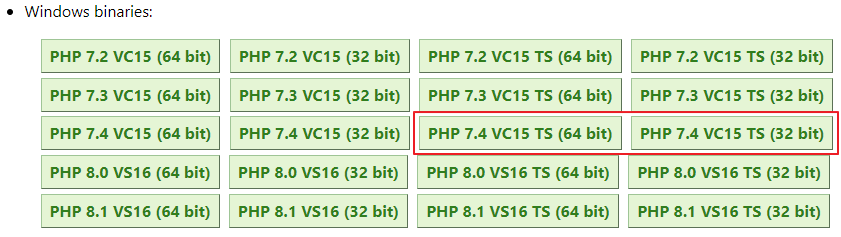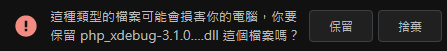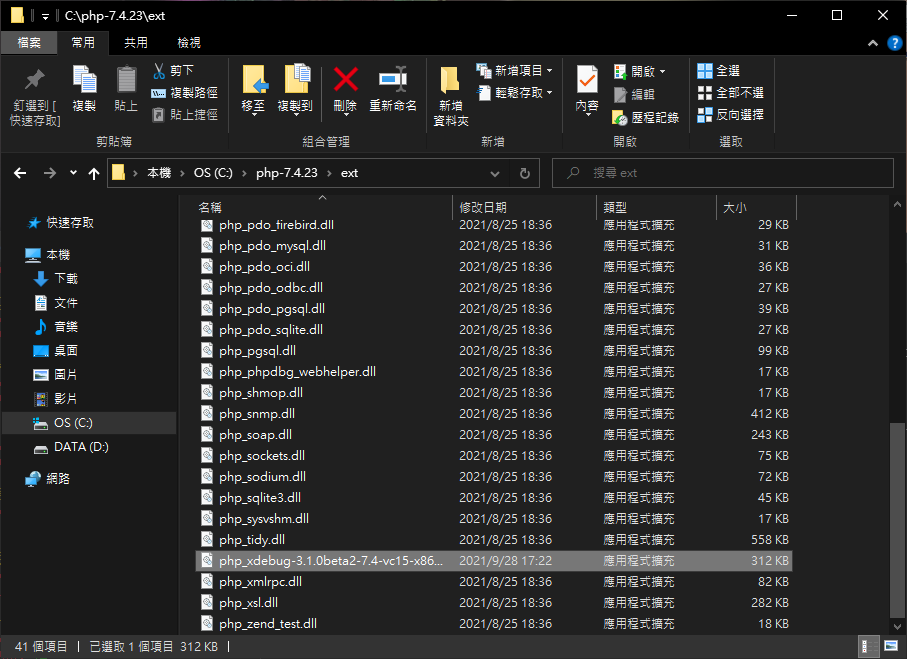[Xdebug]
zend_extension = "path-to-Xdebug.dll"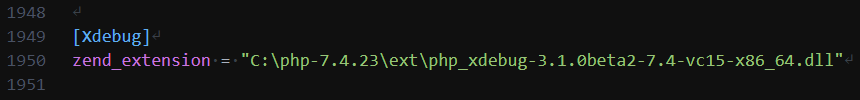使用 Xdebug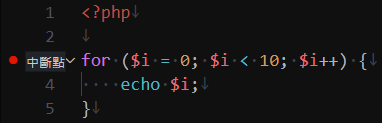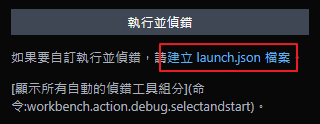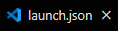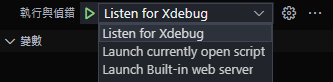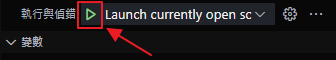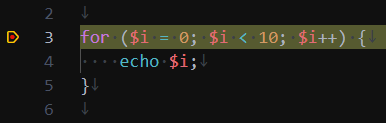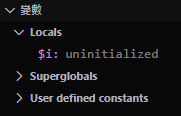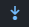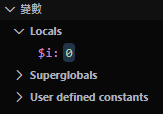Xdebug 功能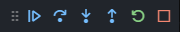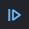繼續執行程式直到下一個斷點，或是程式結束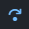執行一步程式，遇到函式就只當做一步，不會跑進函式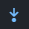執行一步程式，會跑進函式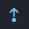直接執行到函式結束，會停在函式後的下一步位置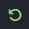重新執行偵錯，從頭開始執行程式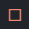中止偵錯，直接停止程式，不再執行